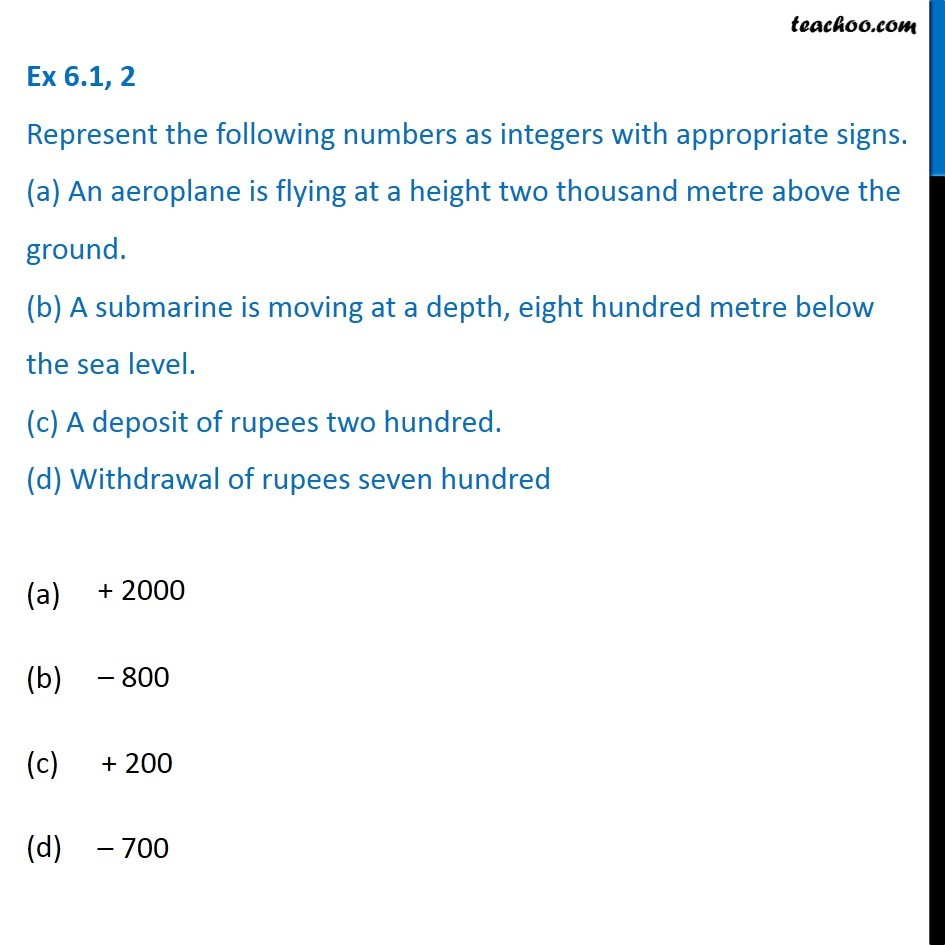1. Chapter 6 Class 6 Integers
2. Concept wise
3. Definitions

Transcript

Ex 6.1, 2 Represent the following numbers as integers with appropriate signs. (a) An aeroplane is flying at a height two thousand metre above the ground. (b) A submarine is moving at a depth, eight hundred metre below the sea level. (c) A deposit of rupees two hundred. (d) Withdrawal of rupees seven hundred+ 2000 800 + 200 700

Definitions

Chapter 6 Class 6 Integers
Concept wise